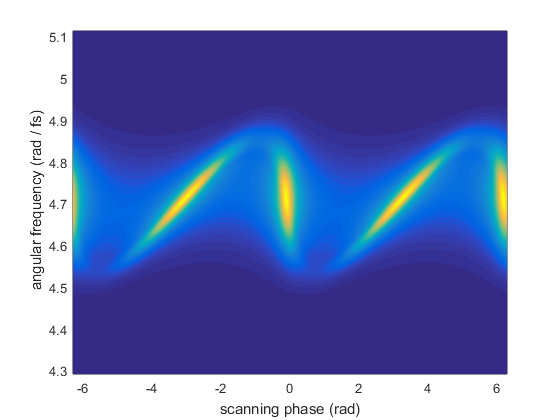# Example of MIIPS simulation

Multiphoton Intrapulse Interference Phase Scan (MIIPS) is a popular technique for femtosecond pulse compression, which involves modulating the spectral phase and recording second harmonic spectra.

The second harmonic generation (SHG) is sensitive to the spectral phase, so by analyzing the dependence of the SHG from the modulation parameters it is possible to derive an estimate of the spectral phase.

## Initialize laser pulse

```p = gaussianPulse('f0', 300/800, 't0', 0, 'fwhm', 20, ...
'dt',1, 'nPoints', 2^12);
p.polynomialPhase([1e4 100 0 0])
```

## Set up the modulation parameters

In the most common implementation of MIIPS, the phase modulation is a sinusoid, given by:

amp * sin( tau * (w - w0) - phi)

where phi is a scanning parameter.

```% modulation amplitude
% modulation frequency
tau = 10; % fs
% scanning parameters
phaseSteps = -2*pi: 0.01 : 2*pi;
```

## Create a matrix with the phase modulation mask

The modulation mask must be a matrix with frequency on the first dimension and scanning parameter on the second dimension.

```[W, PHI] = ndgrid(2*pi * p.frequencyArray, phaseSteps);
W0 = 2*pi * p.centralFrequency;
% the phase modulation mask is:
modulationMask = exp(1i * amp * sin(tau * (W-W0) - PHI));
```

## Calculate the MIIPS trace

Multiply the pulse by the phase modulation mask

```% make sure to multiply in the frequency domain
p.activeDomain = 'frequency';
```

Calculate the second harmonic and get a LaserPulse object which contain the MIIPS trace.

```% harmonics are easier to calculate in time domain
p.activeDomain = 'time';
miips = p^2;
```

## Display the MIIPS trace

```figure()